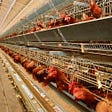# Collecting Weather Data to Boost Data Science Models with Selenium

`import numpy as npimport pandas as pdimport timeimport datetimeimport refrom selenium import webdriver`
`driver = webdriver.Chrome('/usr/local/bin/chromedriver')driver.get('https://www.wunderground.com/history/airport/KSFO/2018/03/14/DailyHistory.html?req_city=San%20Francisco&req_state=CA&reqdb.zip=94128&reqdb.magic=4&reqdb.wmo=99999')`
`def list_dates(start,end):    """ This creates a list of of dates between the 'start' date and the 'end' date """    # create datetime object for the start and end dates    start = datetime.datetime.strptime(start, '%Y-%m-%d')    end = datetime.datetime.strptime(end, '%Y-%m-%d')    # generates list of dates between start and end dates    step = datetime.timedelta(days=1)    dates = []    while start <= end:        dates.append(start.date())        start += step    # return the list of dates in string format    return [str(date) for date in dates]`
`# this dictionary is used to map the months produced in the previous function with the full name of the month     month_dict ={    1:'January',    2:'February',    3:'March',    4:'April',    5:'May',    6:'June',    7:'July',    8:'August',    9:'September',    10:'October',    11:'November',    12:'December'}def date_part(data,f_mat ='%Y-%m-%d'):    """Extracts the date information produced by list_dates() for Month,Day,Year """    # creates a pandas dataframe of dates    dates = pd.DataFrame(data,columns=['date'])    date_time = dates['date']    fld = pd.to_datetime(date_time, format=f_mat)    for n in ('Month', 'Day','Year'):        dates[n] = getattr(fld.dt,n.lower())    dates['Month'] = dates['Month'].map(month_dict)    return dates`
`start = '2014-1-1'end = '2014-1-4'date = list_dates(start,end)date = date_part(date,'%Y-%m-%d')`
`zipcode='91770'search=driver.find_element_by_id('history-icao-search')search.clear() # clears fieldsearch.send_keys(zipcode) # send zipcode to search fieldsearch.submit() # submits zip code to search`
`Month='March'Day='14'Year='2018'month = driver.find_element_by_class_name('month')month.send_keys(Month)day = driver.find_element_by_class_name('day')day.send_keys(Day)year = driver.find_element_by_class_name('year')year.send_keys(Year)year.submit()`
`weatherdata = driver.find_elements_by_id('observations_details').text`
`from selenium import webdriverimport timeimport numpy as npimport pandas as pdimport datetimeimport pickleimport redef list_dates(start,end):    """ This creates a list of of dates between the 'start' date and the 'end' date """    # create datetime object for the start and end dates    start = datetime.datetime.strptime(start, '%Y-%m-%d')    end = datetime.datetime.strptime(end, '%Y-%m-%d')    # generates list of dates between start and end dates    step = datetime.timedelta(days=1)    dates = []    while start <= end:        dates.append(start.date())        start += step    # return the list of dates in string format    return [str(date) for date in dates]# this dictionary is used to map the months produced in the previous function with the full name of the month month_dict ={    1:'January',    2:'February',    3:'March',    4:'April',    5:'May',    6:'June',    7:'July',    8:'August',    9:'September',    10:'October',    11:'November',    12:'December'}def date_part(data,f_mat ='%Y-%m-%d'):    """Extracts the date information produced by list_dates() for Month,Day,Year """    # creates a pandas dataframe of dates    dates = pd.DataFrame(data,columns=['date'])    date_time = dates['date']    fld = pd.to_datetime(date_time, format=f_mat)    for n in ('Month', 'Day','Year'):        dates[n] = getattr(fld.dt,n.lower())    dates['Month'] = dates['Month'].map(month_dict)    return datesdef scrapper(dates,zipcode):    data=[] # list to append scrapped data    # submits the zipcode to find the closest weather center    search= driver.find_element_by_xpath('//*[@id="history-icao-search"]')    search.clear()    search.send_keys(zipcode)    search.submit()    time.sleep(3) # sleep timer to wait for page to load (not necessary)        # iterates through provided list of dates to scrap weather for    for i,v in dates.iterrows():        # inputs month, day, year into website to view information        month = driver.find_element_by_class_name('month')        month.send_keys(v['Month'])        day = driver.find_element_by_class_name('day')        day.send_keys(v['Day'])        year = driver.find_element_by_class_name('year')        year.send_keys(v['Year'])        year.submit() # submits to search for month, day, year#         time.sleep(3) # sleep timer to wait for page to load (not necessary)# scraps table on the bottom for weather information        weatherdata = driver.find_elements_by_id('observations_details') # locates the data        x = weatherdata.text # scrapes that data        x= re.sub(r'[^\x00-\x7F]+',' ', x) # removes unicode        x = x.split('\n') # breaks the data into observations per row        x = x[1:-1] # removes the last line        data.extend([i+' '+v['date'] for i in x]) # appends all scraped data    return datadef preprocess_data(data):    """Preprocess the scraped data and load the data into a pandas dataframe"""    dt = [i.replace('Calm Calm', 'Calm 0.0 mph') for i in data]    dt = [i.replace(' AM', 'AM') for i in dt]    dt = [i.replace(' PM', 'PM') for i in dt]    dt = [i.replace('%', '') for i in dt]       dt = [i.replace(' mi', '') for i in dt]    dt = [i.replace(' mph', '') for i in dt]    dt = [i.replace(' in', '') for i in dt]    dt = [re.sub(' +',' ',i) for i in dt]dt = [i.replace('Mostly ', 'Mostly') for i in dt]    dt = [i.replace('Partly ', 'Partly') for i in dt]    dt = [i.replace('Scattered ', 'Scattered') for i in dt]    dt = [i.replace('Light ', 'Light') for i in dt]    dt = [i.replace('Heavy ', 'Heavy') for i in dt]        dt = [i.replace('Fog , Rain', 'Rain') for i in dt]    dt = [i.replace('Fog , Snow', 'FogSnow') for i in dt]    dt = [i.replace('Fog', ' ',1) for i in dt]    dt = [i.replace('Rain , Thunderstorm', 'RainThunderstorm') for i in dt]        dt = [i.replace('Thunderstorm', '',1) for i in dt]    dt = [i.replace('Thunderstorms and Rain', 'ThunderstormsandRain') for i in dt]dt = [i.replace('Rain', '',1) for i in dt]    dt = [i.replace('Snow', '',1) for i in dt]#     dt = [i.replace('Light Drizzle', 'LightDrizzle') for i in dt]        dt = [i.replace('F', '',) for i in dt]    dt = [i.replace(' og', ' Fog') for i in dt]    dt = [i.replace('Patches of Fog', 'PatchesofFog',1) for i in dt]    dt = [i.replace('Lightreezing Rain', 'LightFreezingRain') for i in dt]      dt = [i.split() for i in dt]    dt = [ i[:2] +i[-10:] for i in dt]        dt = pd.DataFrame(dt,columns = ['time','temp(F)','dewpoint(F)','humidity(%)','pressure(in)','visibility(mi)','winddir','windspeed(mph)','gustspeed(mph)','precip(in)','conditions','date'])    dt['time'] = [datetime.datetime.strftime(datetime.datetime.strptime(val, "%I:%M%p"), "%H:%M") for val in dt['time']]    return dtdef weather_scrapper(start_date,end_date, zipcode):    """final webscrapper function"""    dates = list_dates(start_date,end_date)    dates = date_part(dates,'%Y-%m-%d')    data = scrapper(dates,zipcode)    return preprocess_data(data)####################################################################driver = webdriver.Chrome('/usr/local/bin/chromedriver')x = 'https://www.wunderground.com/history/airport/KSFO/2018/2/24/DailyHistory.html?req_city=San%20Francisco&req_statename=California'driver.get(x)# YYYY-MM-DDstart = '2014-1-1'end = '2014-1-5'zipcode = '10001'weather_scrapper(start,end,zipcode)`

--

--

--

## More from David Kes

Data Scientist | MS in Data Science;

Love podcasts or audiobooks? Learn on the go with our new app.

## Supporting the Sea Ice for Walrus Outlook (SIWO) with an animation of sea ice movement from HYCOM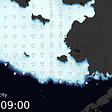## Univariate Statistics in python## Converting a Probability to Fail Into a Time to Failure Metric## Sonification: telling data stories through sound## Predictive Analytic automation tools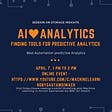## Data Transfer from AWS Redshift to BigQuery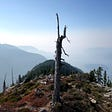## 9 Most Essential Data Science Skill You Should Have in 2022## Research for Design Part 5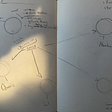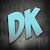## David Kes

Data Scientist | MS in Data Science;

## 2018 Week 35 Aug 27 Makeovermonday dataset : Wearables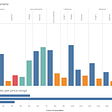## In-Depth Understanding of K-Means Clustering in Machine Learning.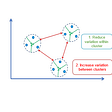## Simple Linear Regression — KNIME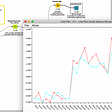## Turning Raw Poultry Farm Data into meaningful insights.